# How to Calculate Compound or Average Annual Growth Rate

This post will guide you how to calculate the compound annual growth rate (CAGR) in excel. How to calculate the average annual growth rate (AAGR) using excel formula.

## What is Compound Annual Growth Rate (CAGR) and AAGR?

CAGR measures the rate of return for an investment over an investment period. It is also called a “smoothed” rate of return because it measures the growth of an investment have grown on an annual basis.

The average annual growth rate (AAGR) is the arithmetic mean of a series of annual growth rates.

## Calculate Compound Annual Growth Rate

To calculate compound annual growth rate, you need to divide the ending value of the investment by the value at the start of the period, then raise the result to the power of one divided by the number of years, and then subtract one form the subsequent result.

If you want to calculate compound annual growth rate, you can try to use the below generic CAGR formula:

`=(EV/BV)^(1/n)-1`

BV – start value of the investment

EV – End value of the investment

N – Number of years

Assuming that you have the start value in Cell B1, the ending value of the investment in cell B2, the number of periods in B3. Then we can write down the following formula to calculate CAGR:

`=(B2/B1)^(1/B3)-1`

1# enter the above formula into cell C22# select the cell you entered the formula (Cell C2), then Go to HOME Tab, click “Percent Style” command under Number group to convert the number to percentage format.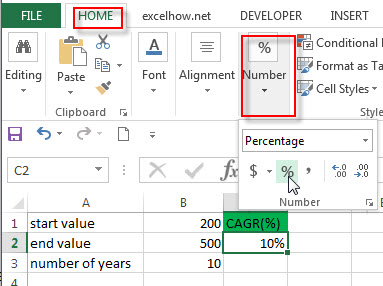You can also calculate compound annual growth rate using the POWER function to create the following generic excel formula:

`=POWER(EV/BV,1/n)-1`

For above example, you can use this generic formula to write down the following formula:

`=POWER(B2/B1,1/B3)-1`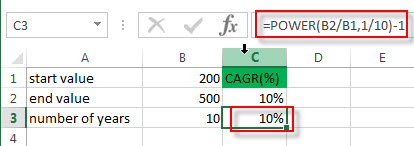There is another method for calculating CAGR in excel, you can use the RATE function to create the following generic formula:

`=RATE(n,,-BV,EV)`

N is the number of years

Bv is the beginning value of the investment

EV is the ending value of the investment

So you can enter the below formula in the Cell C2 to calculate CAGR:

`=RATE(B3,,-B1,B2)`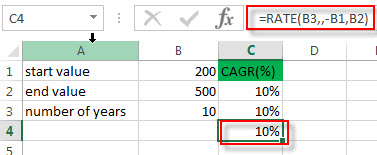## Calculate  Average Annual Growth Rate (AAGR)

You can use the below generic formula to calculate the average annual growth rate in excel.

`AAGR=(Growth rate A + Growth rate B +…)/number of years`

Assuming that you have a list of revenues for a 5-year period, you need to get the growth rate of each year firstly, such as, calculating the growth rate from 2013-2014 is (B3-B2)/B2=20%. So the generic formula of growth rate is as follows:

`Growth rate = (Ending value – Beginning Value)/ Beginning value`

And then you need to average those annual growth rates to get the AAGR value.

Let’s refer to the following steps to calculate CAAG:

1# enter the above formula of growth rate into the cell C3 to the growth rate in 2014, then press Enter key. Then drag the AutoFill Handle down to other cells.

`=(B3-B2)/B2`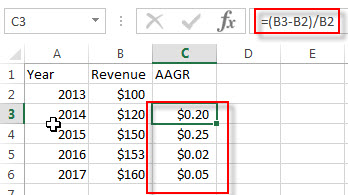2# select the range C3:C6, then go to Home Tab, click “Percent Style” command under Number group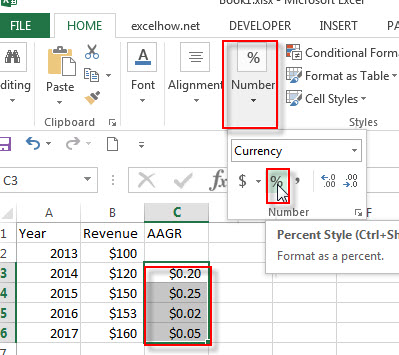3# you will see that you have the 4 annual growth rates for a 5-year period in C3:C6.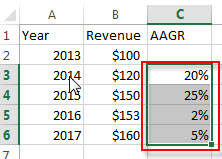4# next you need to use the AVERAGE function to average all annual growth rates to get the AAGR. So enter the below formula in Cell C7.

`=AVERAGE(C3:C6)`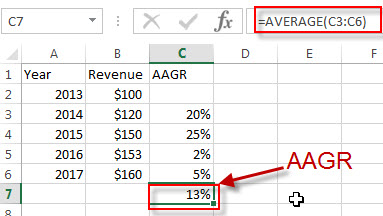### Related Functions

• Excel AVERAGE function
The Excel AVERAGE function returns the average of the numbers that you provided.The syntax of the AVERAGE function is as below:=AVERAGE (number1,[number2],…)….
• Excel RATE function
The Excel RATE function returns the interest rate per payment period of an annuity.The syntax of the RATE function is as below:=RATE(nper, pmt,pv,[fv],[type],[guess])….